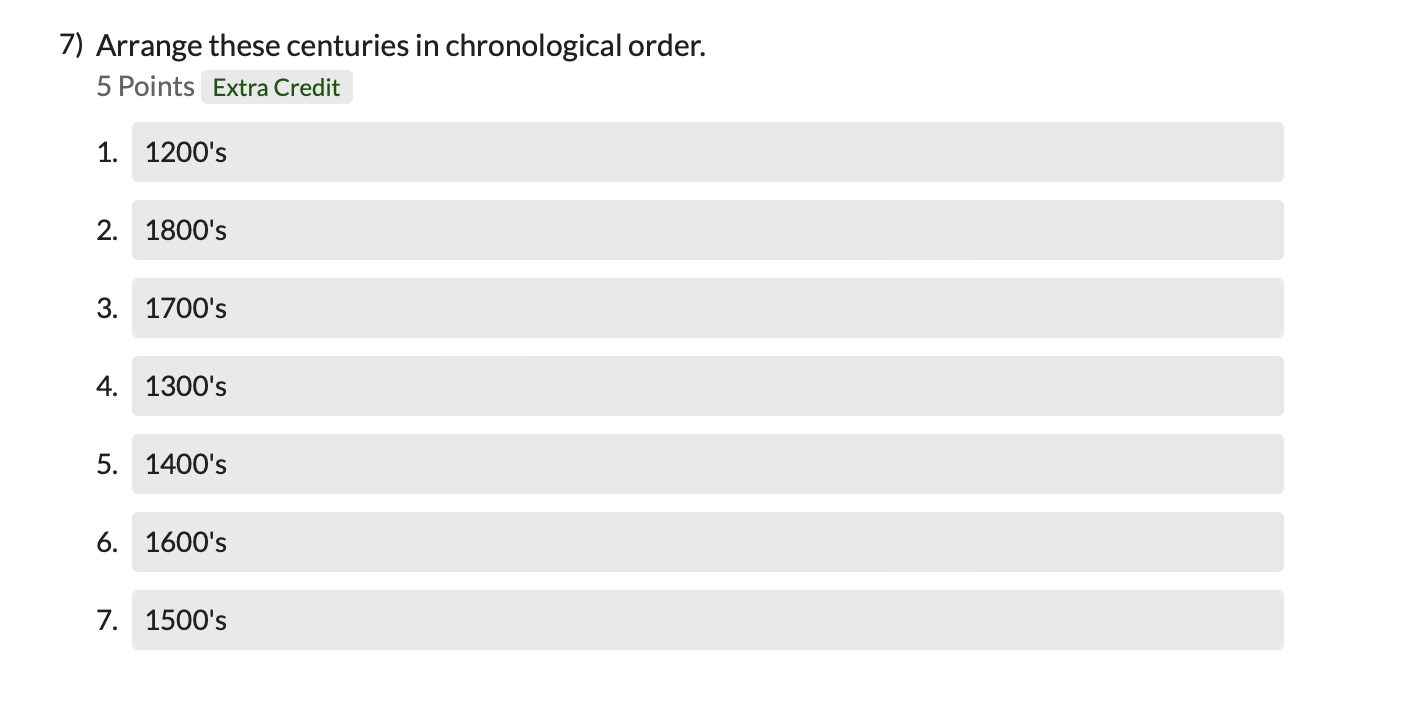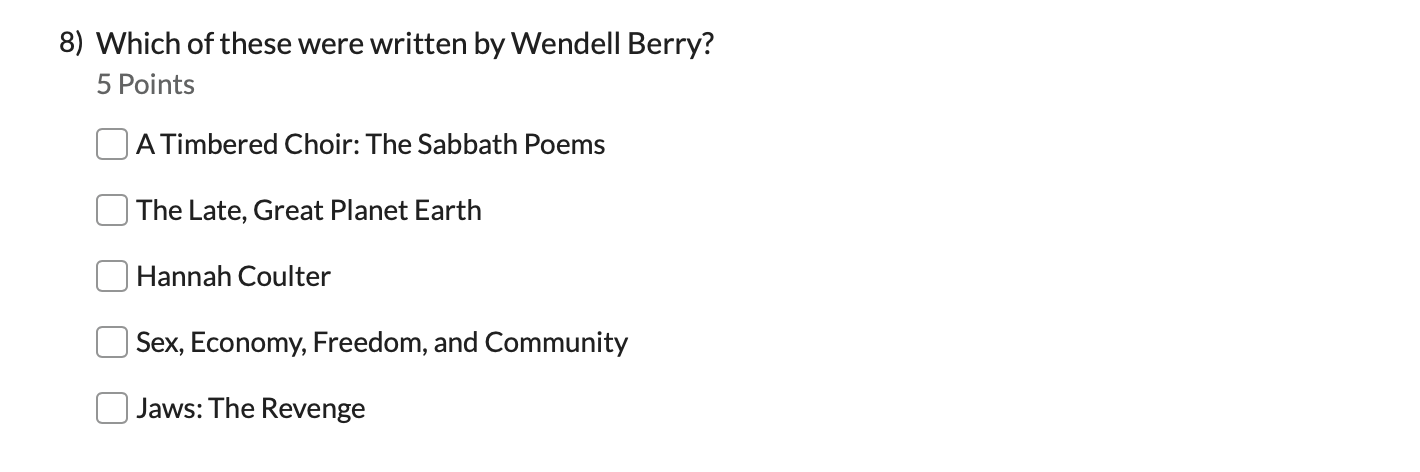Follow

# How does partial credit work in online test questions?

Partial credit is available in some form for each answer type. You can set up your questions so that Populi will automatically assign partial credit for certain answers; you can also give partial credit if you're grading answers manually.

Remember that if you don't check Partial Credit on a given question, your students will need to enter the correct answer—no more, no less—to receive credit for that question.

Here's how Populi approaches automatic partial credit for put in order, multiple answer, and matching questions:

### Put in OrderPartial credit for put-in-order questions is really tricky. Awhile back, we described Populi's process in detail on the Populi Blog.Multiple answer checks which answers the student marked/didn't mark. If he marks (or doesn't mark) something correctly, he gets a portion of the question's point value. If he does so incorrectly, that portion of the question's point value is deducted from his score.

Put another way, an incorrect option cancels out a correct option.

In this example, a 5-point question has five options, three of which are correct. Here's how it would be scored:

 Correct √ Student Points A √ √ +1 B √ -1 C √ -1 D √ √ +1 E +1

So the student, having marked three options correctly and two incorrectly ends up with 1/5 of the question's point value: the two incorrect selections cancel out two of the three correct selections.

### MatchingMatching questions act much the same as multiple answer: the student simply has to match the correct terms together.

 Correct Student Credit? A A Yes B F No C B No D D Yes E E Yes

So the student, having matched 3 of 5 answers, gets 3/5 of the credit. Pretty straightforward.